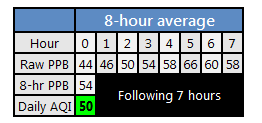# Daily and Hourly AQI - Ozone

## Daily Ozone AQI

The daily ozone AQI is calculated by taking the maximum 8 hour concentration from 07:00-23:00 LST, and then converting to AQI. Each 8-hour average concentration is truncated to .001ppm/1ppb. There are 17 8-hour averages considered in each day. Each 8-hour average requires 6 of 8 hours (75%) for a valid calculation. In addition, 75% or 13/17 of the 8-hour averages are needed for a valid daily ozone AQI calculation.Example 1: Valid

Example 2: Valid with Missing Data (Red background/text= invalid or missing)

Example 3: Insufficient Data (Red background/text= invalid or missing)

## Hourly Ozone AQI

If viewing/calculating hourly ozone AQI for hours >1 old, these calculations are done using the midpoint 8-hour average ozone concentration which is then converted to AQI. For a given hour, this includes the previous 4 hours, the given hour, and the following 3 hours. 6 of 8 hourly concentrations (75%) are needed for a valid midpoint 8-hour average.Example 1: No Missing Data

Example 2: Some Missing Data